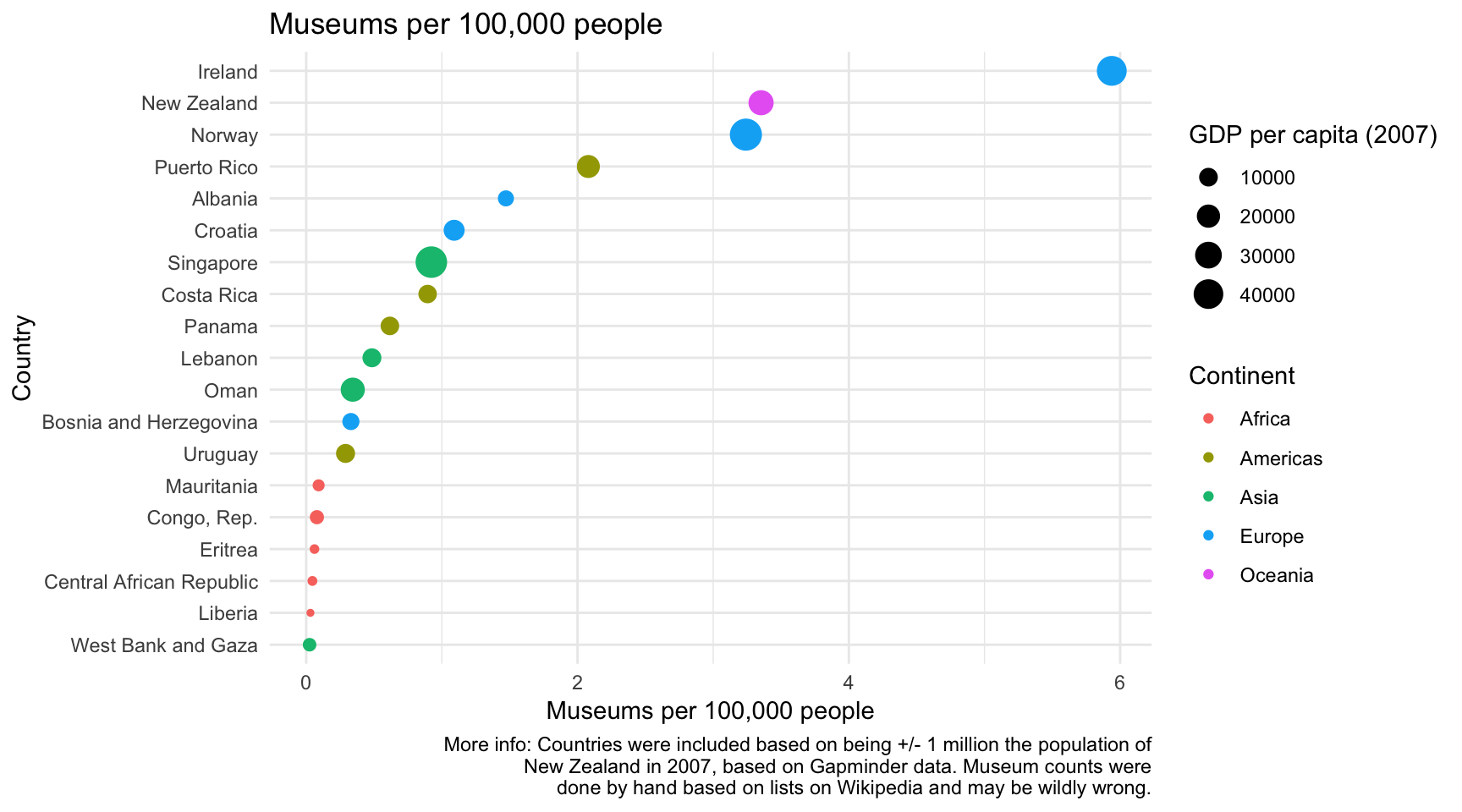# A quick look at museums per capita

I saw a cool tweet from Scott Pilkington today about museums per capita, and Aimee Whitcroft had some interesting follow up questions about countries similar in population to New Zealand…this was far too good a procrastination opportunity!

<!DOCTYPE html>

Museums per capita
library(tidyverse)
library(rvest)
library(gapminder)

# Getting some comparison countries

I decided to grab some countries with populations within a million of New Zealands. The easiest dataset I new with loads of population data is in the lovely gapminder package. Unfortunately the most recent population counts are for 2007, but this attempt is so back-of-the-envelope anyway, and more just a chance to play, so I’m going to just go with that. Also provides GDP per capita which I think is interesting for arts and culture funding.

my_pop = gapminder %>%
filter(year == max(year)) %>%
mutate(NZpop = ifelse(country == "New Zealand", pop, NA)) %>%
mutate(NZpop = as.numeric(NZpop)) %>%
arrange(NZpop) %>%
fill(NZpop) %>%
mutate(Difference from NZ = pop-NZpop) %>%
filter(abs(Difference from NZ) < 1000000)

# Examples of getting number of museums from Wikipedia lists

Below are a couple very messy examples of scraping the individual pages on Wikipedia:

NZ = read_html("https://en.wikipedia.org/wiki/List_of_museums_in_New_Zealand") %>%
html_nodes("ul") %>%
html_text() %>%
as.data.frame() %>%
.[3:22,] %>%
as.data.frame() %>%
rename(name = 1) %>%
separate_rows(name, sep = "\\\n")

nrow(NZ)
##  138
ireland = read_html("https://en.wikipedia.org/wiki/List_of_museums_in_the_Republic_of_Ireland") %>%
html_nodes("table") %>%
.[] %>%
html_table(fill = TRUE)

nrow(ireland)
##  244
norway = read_html("https://en.wikipedia.org/wiki/List_of_museums_in_Norway") %>%
html_nodes("ul") %>%
html_text() %>%
as.data.frame() %>%
.[3:22,] %>%
data_frame() %>%
rename(name = 1) %>%
separate_rows(name, sep = "\\\n")
nrow(norway)
##  150
oman = read_html("https://en.wikipedia.org/wiki/List_of_museums_in_Oman") %>%
html_nodes("ul") %>%
html_text() %>%
as.data.frame() %>%
.[1,] %>%
data_frame() %>%
rename(name = 1) %>%
separate_rows(name, sep = "\\\n")
nrow(oman)
##  11
panama = read_html("https://en.wikipedia.org/wiki/List_of_museums_in_Panama") %>%
html_nodes("table") %>%
.[] %>%
html_table(fill = TRUE)
nrow(panama)
##  20
puertorico = read_html("https://en.wikipedia.org/wiki/List_of_museums_in_Puerto_Rico") %>%
html_nodes("table") %>%
.[] %>%
html_table(fill = TRUE)
nrow(puertorico)
##  82
singapore = read_html("https://en.wikipedia.org/wiki/List_of_museums_in_Singapore") %>%
html_nodes("ul") %>%
html_text() %>%
as.data.frame() %>%
.[2:4,] %>%
data_frame() %>%
rename(name = 1) %>%
separate_rows(name, sep = "\\\n")
nrow(singapore)
##  42
uruguay = read_html("https://en.wikipedia.org/wiki/List_of_museums_in_Uruguay") %>%
html_nodes("ul") %>%
html_text() %>%
as.data.frame() %>%
.[1,] %>%
data_frame() %>%
rename(name = 1) %>%
separate_rows(name, sep = "\\\n")
nrow(uruguay)
##  10

The ideal would be to use the main page to get all the hyperlinks with something like this:

get_links = read_html("https://en.wikipedia.org/wiki/List_of_museums_by_country") %>%
html_nodes("a") %>%
html_attr("href") %>%
data.frame() %>%
mutate(links = paste0("https://en.wikipedia.org/", links))

…and then scrape each of these, but aside from there being several ways these pages are formatted, you also have some lists just under the country title, not linking to a new page, and then you have some missing (I think Republic of Congo’s list of museums is missing from this main page). Ugh.

# Making a graph

So here is a quick look at the number of museums per 100,000 people. There are quite a few limitations on the data of course, but interesting none the less, I hope.

# numbers mostly by hand from wikipedia

tidy_museums = museums %>%
mutate(Museums per 100,000 = N museums from Wikipedia/pop*100000) %>%
filter(!is.na(country))

tidy_museums %>%
mutate(country = fct_reorder(country, Museums per 100,000)) %>%
ggplot(aes(x = Museums per 100,000, y = country, size = gdpPerCap, color = continent)) +
geom_point() +
scale_size_continuous(name = "GDP per capita (2007)") +
scale_color_discrete(name = "Continent") +
ylab(label = "Country") +
xlab(label = "Museums per 100,000 people") +
theme_minimal() +
ggtitle("Museums per 100,000 people") +
labs(caption = "More info: Countries were included based on being +/- 1 million the population of\nNew Zealand in 2007, based on Gapminder data. Museum counts were\ndone by hand based on lists on Wikipedia and may be wildly wrong.")Code and data available at https://github.com/elb0/museums-per-capita.

Written on March 26, 2019

Explore all tags: event-notes book-recommendations R-code PhD social-media event-planning